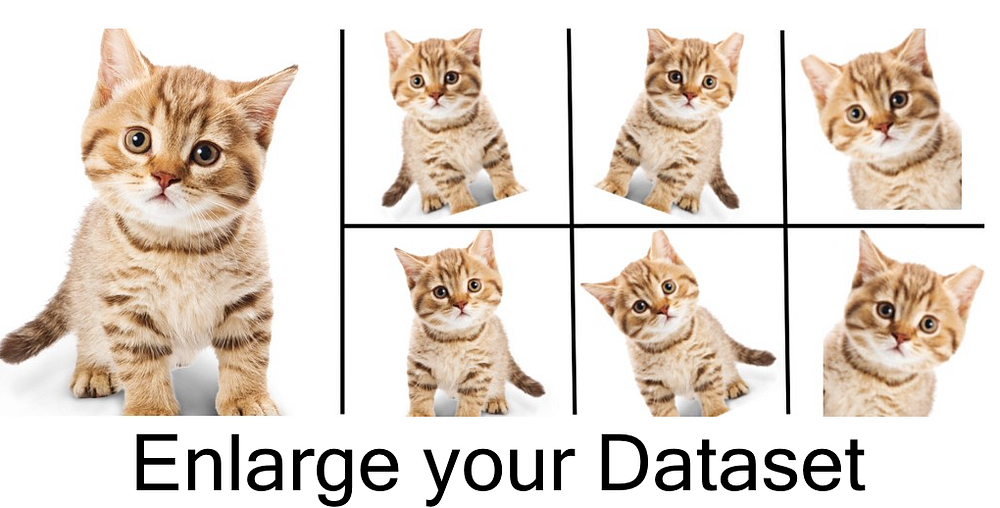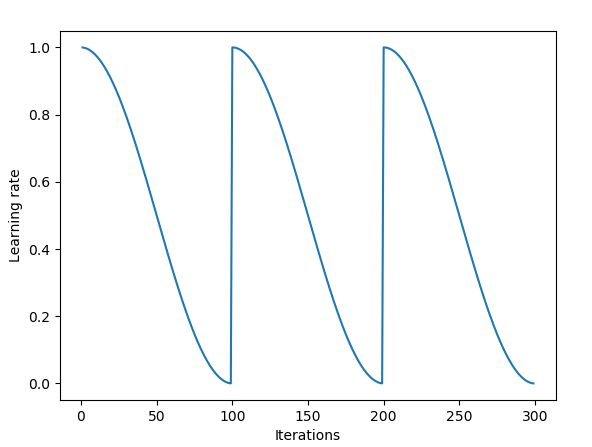Fast.ai Deep Learning Part 1 — Lesson 1 My Personal Notes.

Summary: In this lecture we dive deeper to image classification. We learn state of the art techniques which makes the classifier a lot better. Most of these techniques are not very popular although with these things you can easily get into top 10 in Kaggle competitions.

Codes: First, Second, Third

Video

First code!

[15:00]

The most important thing you can do, to make your model better, is giving more data. With a small amount of data model often over fit (we learn later what this mean) and not work well with test set data. Getting more data doesn’t mean that you have to collect new images and label those. Data augmentation is a technique which modify images in your data set producing new images. This is a great way to get as much as plausible out of your data set. Often people can spend weeks collecting data when they could get same or even better result with data augmentation.

Many courses doesn’t teach this and that is silly because this is one of the most important things to make model better. Data augmentation zooms in to random places. It also rotate the picture to make it more general. From one image data augmentation can make tens of images which all are little different. You might think why small zooming or rotating change anything. Well when models are training parameters it is important model to understand that cat is not always same place or same size.

It is also important to notice that not all data can be augmented same way. For example, digits can’t be flipped horizontally because the meaning is not anymore the same. Also we can’t flip cat pictures vertically because cats are not normally upside down. But if we are looking satellite pictures rotating all directions is plausible. Often it is good to print out the pictures after data augmentation so you can see that there is not cats upside down.

Data augmentation in fast.ai

`tfms = tfms_from_model(resnet34,sz,aug_tfms=transforms_side_on,max_zoom=1.1)Second code!data = ImageClassifierData.from_paths(PATH,tfms=tfms)`

When we called the function `learn.fit(1e-2,3)` we got this output.

`epoch      trn_loss   val_loss   accuracy                          0      0.082848   0.023114   0.992         1      0.042392   0.026235   0.9915                            2      0.039483   0.029074   0.988                         `

The first two columns are `trn_loss` and `val_loss` . Later in this course you will learn how loss is calculated but for now it is just a number which identify how wrong the predictions are. Thing you have to know is that if train loss is less than validation loss then the model is over fitted and data augmentation might help. Your model can also over fit if you run too many epochs.

`fit` function take also third parameter which is cycle_len. What cycle_len do? It is using a technique called stochastic gradient descent with restarts (SGDR). Idea is that when the parameters are getting closer and closer to the optimal the learning rate become smaller. But then every time at some point close to the optimal we make our learning rate big again. This method makes gradient to find a place which generalize well.

So if parameters start from right corner. They first go down to the closest minimum. Then it increase the learning rate and the gradient jumps to other valley left side. Left valley is better because it is wider which mean it is generalizing better. This trick is quite new and not very popular but Jeremy said that this is one of the reasons why he win Kaggle competitions.First learning rate is a big number then it decrease until some point it is set back to beginning.

[44:00]

Currently we have used pretrained models and we have not changed the parameters of these models. All we have done is added one layer at the end so we have been available use the pretrained models. We can start changing the parameters with command `learn.unfreeze().` Because we don’t want to change later layers so much we can give own learning rate to every layer `lr=np.array([1e-4,1e-3,1e-2]).` This is also very powerful and unpopular technique to make your model better. First unfreeze the pre-trained model and then give those layers small learning rate so you don’t change those too much. There is also parameter called `cycle_mult=2` which mean that every epoch it is decreasing learning rate slower.

[1:10:21]

Every year the libraries change so it is important to understand the concepts. Fast.ai library make it easier to build models and that why it is good for beginners. Later when you start knowing these stuffs you probably want to use Pytorch in some cases but you can also then use Fast.ai to make it easier to write some methods.

This image classifier is now getting the state of the art results. Let’s recall the steps:

1. Enable data augmentation, and precompute=True
2. Use lr_find() to find highest learning rate where loss is still clearly improving.
3. Train last layer from precomputed activations for 1–2 epochs.
4. Train last layer with data augmentation (i.e. precompute=False) for 2–3 epochs with cycle_len=1
5. Unfreeze all layers
6. Set earlier layers to 3x-10x lower learning rate than next higher layer
7. Use lr_find() again
8. Train full network with cycle_mult=2 until over-fitting

Second code!

[1:18:00]

Next Jeremy go through the code which is predicting dog’s breed in the image.

• First import data to the code.
• Look some examples from the data to see what kind of data there is. `label_df.pivot_table(index='breed',aggfunc=len)` <- see how many examples there is in each category. You can also print one image, size of it, and other relevant information.
`size_d = {k:PIL.Image.open(PATH+k).size for k in data.trn_ds.fnames}row_sz,col_sz = list(zip(*size_d.values()))row_sz=np.array(row_sz);col_sz = np.array(col_sz)row_sz[:5]`
`# First five images row size.OUTPUT: array([500,500,400,500,231])plt.hist(row_sz)OUTPUT:`
• Data augmentation.
• Take validation set from training data.
• Build model (precompute=True)
• fit it (got 84% accuracy)
• `learn.precompute=False`
• `cycle_len=1`
• `learn.set_data(get_data(299,bs))` This will use the same model that you used with smaller image size but change it to work with 299 image size. This is again the state of the art methods which is not used a lot.
• `cycle_mult=2`
• Finally we got about 94% accuracy which is amazing, because we had 120 different classes.

Third code!

[1:48:55]

Architecture mean how layers are put together. Different architectures can have different numbers of layers in different orders.